#Function Repository Resource:

# Erfci

Set up a symbol to give an error message when called with an unexpected number of arguments

Contributed by: Paco Jain (Wolfram Research)
 ResourceFunction["Erfci"][x] computes the definite integral of Erfc[x].

## Details and Options

Mathematical function, suitable for both symbolic and numerical manipulation.
The ResourceFunction["Erfci"] function is defined through Integrate[Erfc[t],{t,0,x}]+C, where C=-1/Sqrt[π] is an integration constant. In closed form this is equivalent to Exp[-x^2]/Sqrt[π] + x Erfc[x].
ResourceFunction["Erfci"] has the attributes Listable and NumericFunction.
ResourceFunction["Erfci"] can be evaluated to arbitrary numerical precision.

## Examples

### Basic Examples

Plot the Erfci function:

 In:=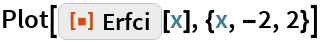Out=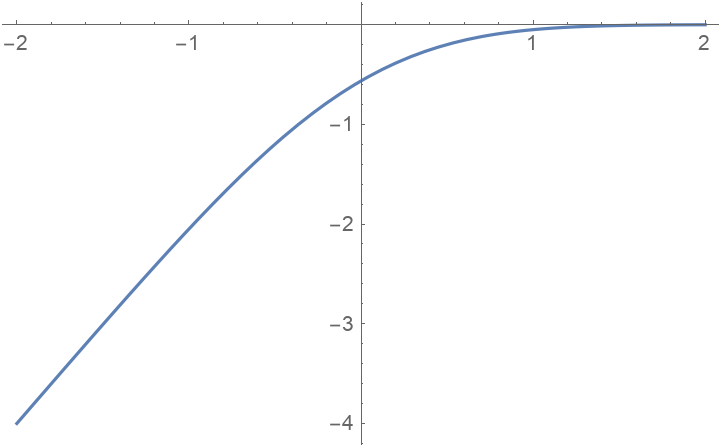Examine some particular values:

 In:=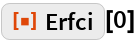Out=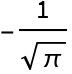In:=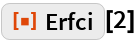Out=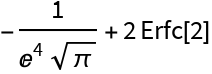### Scope

Erfc can be applied to complex values:

 In:=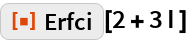Out=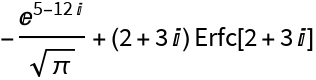In:=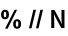Out=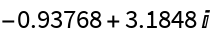When applied to numerical values, Erfc reflects the precision of its input:

 In:=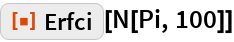Out=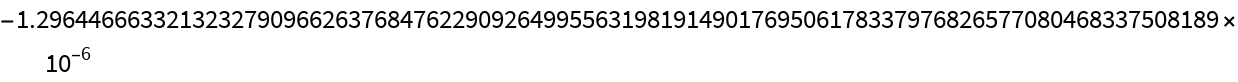## Requirements

Wolfram Language 11.3 (March 2018) or above